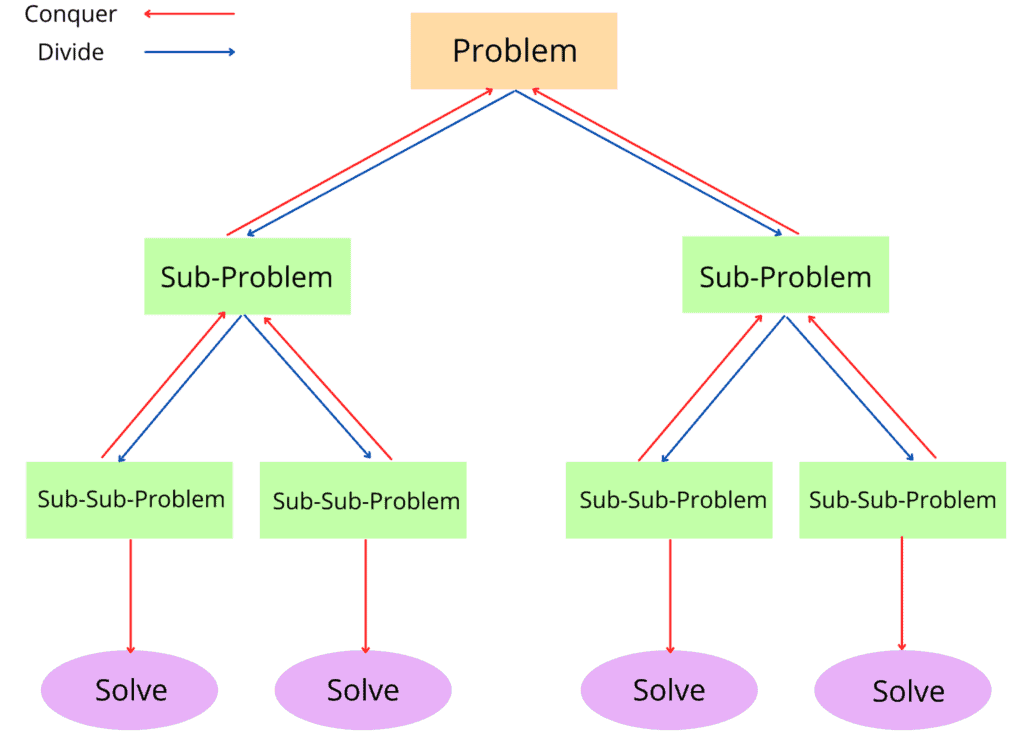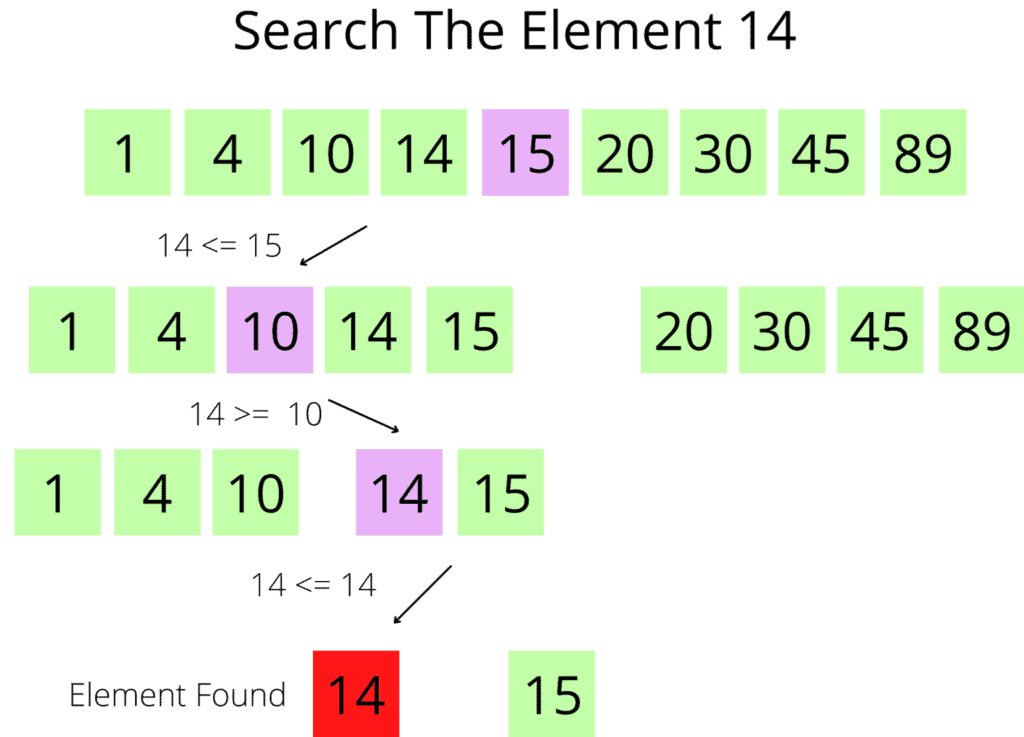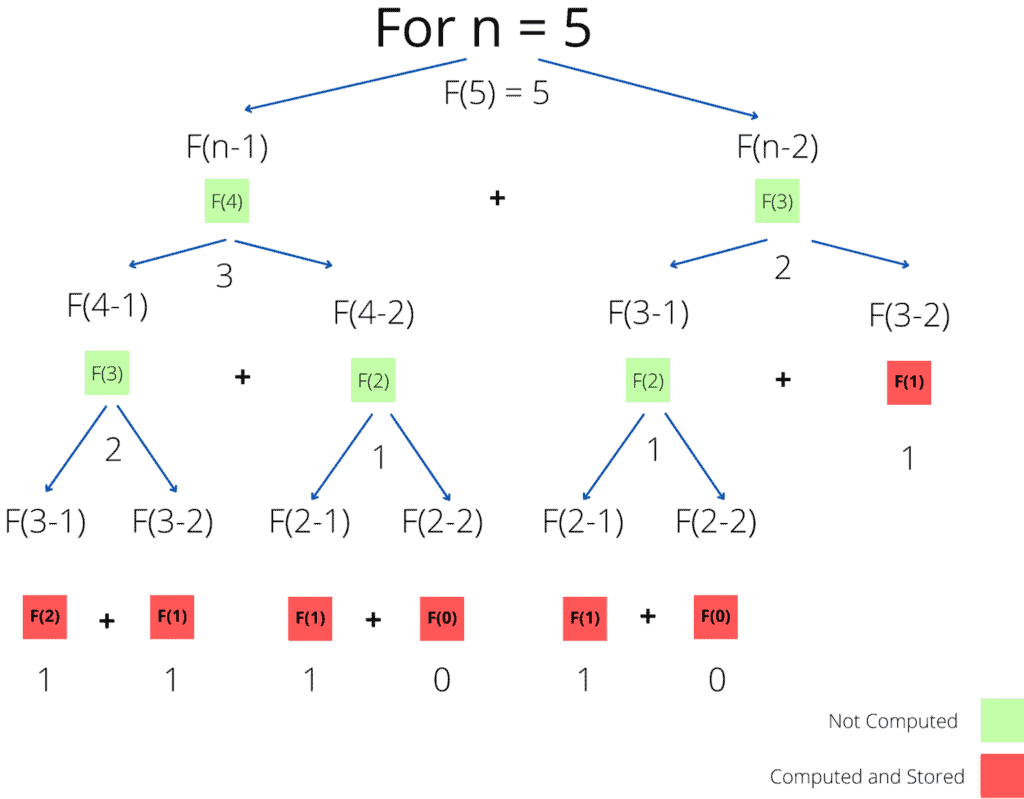## 1. Overview

In this tutorial, we’ll discuss two very popular algorithmic paradigms: divide and conquer and dynamic programming.

We’ll start with the basic idea, followed by an example for each paradigm. Finally, we’ll present the core differences between them.

## 2. Divide and Conquer Approach

The expression “Divide and Conquer” is well-known in politics and sociology, originally used by Roman rulers as “Divide et impera”. It’s a strategy that focuses on dividing elements into sub-elements in order to affect the power and difficulty.

Inspired by this idea, researchers designed the divide and conquer approach. The first phase of a divide and conquer algorithm is to divide or break the main problem. The main idea of the first phase is to divide a given problem into smaller instances or sub-problems.  We divide each sub-problems further into smaller sub-problems until we reach a phase where no more divisions are feasible.

The second phase of a divide and conquer algorithm is solving the sub-problems generated in the first phase. This stage involves solving a lot of sub-problems recursively. However, sometimes we don’t need to solve the sub-problems as they’re marked as solved on their own after the divide phase.

The final phase of a divide and conquer algorithm is to merge the solutions of the sub-problems. The solutions of the sub-problems are merged recursively until we reach a stage when we get a solution to the original problem:Divide and conquer algorithms help considerably reduce the time complexity of solutions.

## 3. Divide and Conquer Algorithm Example

There are several applications of the divide and conquer paradigm, such as binary search algorithm, sorting algorithms.

Here, we present a binary search algorithm to explain how a divide and conquer algorithm practically works. Given an input array of numbers, we need to find whetheris present in the input array or not. The algorithm will return TRUE if it finds the numberin the input array, FALSE otherwise. Additionally, we assume the input array is sorted in this example.

Following the divide and conquer paradigm, we divide the input array into two sub-arrays. Furthermore, we continue diving the sub-arrays into smaller instances until we reach an instance that cannot be divided further. In this example, the elementis present in the input array. Hence, the algorithm returns TRUE as output:The crucial part is we don’t have to visit the whole input array. We visit only a part of the input array to find the solution to the original problem. It reduces the time complexity to.

## 4. Dynamic Programming Approach

Dynamic programming is a popular algorithmic paradigm, and it uses a recurrent formula to find the solution.  It is similar to the divide and conquer strategy since it breaks down the problem into smaller sub-problems.

The major difference is that in dynamic programming, sub-problems are interdependent. Therefore, we store the result of sub-problems and use them in future references for similar or overlapping sub-problems to reduce the execution time.

Hence, dynamic programming is a good choice when the main problem can be divided into smaller sub-problems, and the smaller sub-problems are overlapping in nature. Specifically, dynamic programming optimizes the recursive calls that occur in the divide and conquer approach.

Before computing the solution of a current sub-problem, we examine the previous solutions. If any of the previously computed sub-problems are similar to the current one, we use the result of that sub-problem. Finally, we merge the solution of sub-problems to reach the solution of the original problem.

## 5. Dynamic Programming Algorithm Example

In this section, we’re taking the Fibonacci number problem, and we’ll discuss how it can be solved using the dynamic programming approach. The Fibonacci function can be expressed mathematically as follows:Here,is the user input. To illustrate how the Fibonacci function can be solved using the dynamic programming approach, we’re taking the input value. In the first phase, we divide the original problem into sub-problems. Unlike divide and conquer, it computes the sub-problems iteratively and stores the solutions for future use.

Finally, we combine the solutions of the sub-problems to achieve the solution of the main problem:## 6. Divide and Conquer vs Dynamic Programming

Now we know the theoretical idea behind both the algorithemic paradigms. In some way they are similar but there are difference in their approaches as well. In this section, we’ll summarizes all the previous discussions and enumerate the main differences in a table:## 7. Conclusion

Despite the resemblance of these two strategies, they remain different, with particular uses.

While both approaches help reduces the solution’s complexity, sometimes they can do the opposite as well. So it remains the mission of the researcher to wisely choose the right strategy to apply to solve a problem.

In this tutorial, we discussed the basic idea of divide and conquer and dynamic programming paradigms. Finally, we presented an example of each approach and enumerated the main differences in a table.